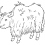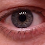## Friday, July 29, 2005

### Math Problem

Saw this on Bash today:
Theorem: All numbers are equal.
Proof: Choose arbitrary a and b, and let t = a + b. Then
a + b = t
`0: a + b = t1: (a + b)(a - b) = t(a - b)2: a^2 - b^2 = ta - tb3: a^2 - ta = b^2 - tb4: a^2 - ta + (t^2)/4 = b^2 - tb + (t^2)/45: (a - t/2)^2 = (b - t/2)^26: a - t/2 = b - t/27: a = b`
So all numbers are the same, and math is pointless.
I went over it twice, and I couldn't find the error.

Let's go through it again, with actual numbers.
Let a=3, b=5. Then, t=8
`0: 3 + 5 = 8» 8 = 8Multiply both sides by (3 - 5) [-2]1: (3 + 5)(3 - 5) = t(3 - 5)» -16 = -16FOIL (no value change)2: 3^2 - 5^2 = 8(3) - 8(5)» 9 - 25 = 24 - 40» -16 = -16Add 5^2 and subtract 8(3) from both sides [+25-24=+1]3: 3^2 - 8(3) = 5^2 - 8(5)» 9 - 24 = 25 - 40» -15 = -15Add (8^2)/4 to both sides [+16]4: 3^2 - 8(3) + (8^2)/4 = 5^2 - 8(5) + (8^2)/4» 9 - 24 + 64/4 = 25 - 40 + 64/4» 1 = 1Factor (no value change)5: (3 - 8/2)^2 = (5 - 8/2)^2» (-1)^2 = (1)^2`      Ahhh...`Root both sides6: 3 - 8/2 = 5 - 8/2» 3 - 4 = 5 - 4» -1 = 1`      Umm...`Add 8/2 from both sides [+4]7: 3 = 5`      Eep.

So wonder_yak is correct, the problem is with rooting both sides. Step 6 should be:
`6: ±(3 - 8/2) = ±(5 - 8/2)`
Isn't math fun?1.Well, I'm not quite sure how to articulate this, but aren't you essentially disregarding sign...specifically when you square and then square-root the equation?

I'm pretty sure that is why this is false, I just wish I knew the algebraic rule that was being broken.

_kris

2.Or, more specifically: in lines 5 - 7 when you factor and then square-root...

3."Isn't math fun?"

Um. See my post on Friday, most notably, the third section.

4.if i tried to read and understand that post.... i would get a headache

5.Not too hard, space cadets. If A not equal to B then at step 5 one expression has to be negative and the square root of a negative is not allowed.

6.Wow, blogboy. Did you even look at step 5? Both sides are squared. The square of any number (negative or positive) is positive. No one is rooting negative numbers. The problem isn't rooting a negative, it's assuming that a root results in a positive.

You fail it.

7.8.